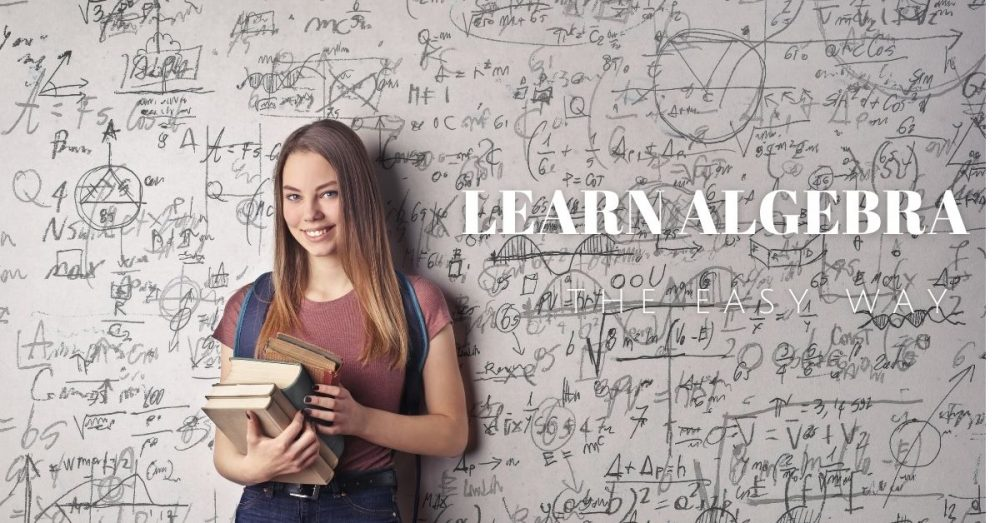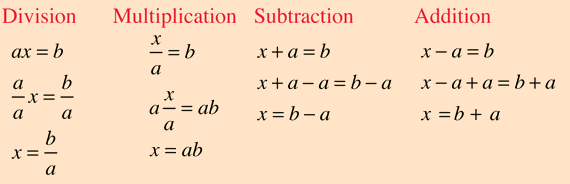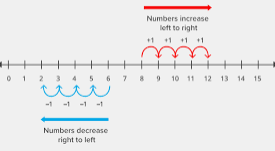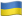# Your Comprehensive Guide to Learning Algebra Fast

Students find algebra one of the most complex subjects in both high school and college because it requires a comprehensive understanding of all the basics of mathematics, including multiplications, subtractions, additions, and divisions. And it is not just algebra, even trigonometry; its close “sister”, is also challenging.No matter the way you look at it, learning algebra looks very complicated, but we are here to demystify the process. So, if you are wondering, “How can I learn algebra,” keep reading as we reveal the secrets that you can use to master this subject and solve eve the toughest equations like an expert.

## Learn Algebra Fast: The Key Points

1. Always understand and utilize the right order of mathematics operations based on the provided acronym: PEMDAS.
2. To make college algebra easy and enjoyable too, you need to be aware of the negative numbers and understand the best way to manage them. While still at this, you need to appreciate that the higher a figure is, the further it is from zero.
3. To make algebra easy, you need to ensure all equations are properly organized.
4. Try to understand the variables that are impossible to define as numbers, such as X, Y, and Z.
5. When solving equations in algebra, try to exclude the number to get only the variables.

Now that we have looked at the main points when learning basic algebra, it is important that we dig deeper into more details. So, if you have Algebra homework to complete, you might be wondering, what exactly is PEDMAS? This is an instrument for helping you to memorize the order of operations. Here is the order:

1. Parenthesis.
2. Exponents.
3. Multiplications.
4. Divisions.
6. Subtractions.

This order is so important and using the wrong one is likely to impact the answer negatively. We will take an example, such as 5+5×4. In this case, if you add 5 to 5 before multiplication, the answer will be 40, which is the wrong answer. The right way for every student to do it in mathematics is multiplying first before addition. So, you should start with 5×4 and then add to get 25, which is the correct answer.

### The principles/ Basics of Learning Basic Algebra

The best way to learn algebra is ensuring to master the basis of arithmetic; including subtracting, adding, multiplying, and dividing that are taught in elementary school. So, we hope you do not have a problem with them, right? The truth about algebra is that it is based on arithmetic basics, but the operations are more complex.
If you are not conversant with the skills we have listed above, the good thing is that there is an easy way to learn algebra online. So, here are the basics of learning algebra.

• In Algebra, Letters are Used to Represent Numbers

If you take the equation such as ax 2+ bx +c=0, letters a, b and c are used to represent known constants that are referred to as coefficients. In the same equation, x is an unknown variable with a value that depends on a, b and c.

• Most Algebra Equations Involve Getting Solutions to Equations

In most algebra equations, the goal is getting solutions to equations, such as finding roots or values of unknowns. For example, x =−2 in algebra is a root of x 2−2 x −8=0 since (−2) 2−2(−2)−8=4+4−8=0. So, substitution will show that x=4 is also a root of the equation.

• The Equations Of Algebra Involve Polynomial Functions.

The Fundamental Theorem of Algebra indicates that each polynomial f ( x )= a n x n + a n −1 x n −1+ … + a 1 x + a 0 that contains a n ≠0 and n ≥1, has at least one complex root, from which follows that the equation f ( x )=0 contains exactly n roots, which can be real or complex and might not all be distinct. To simplify this, let us take an example: x 4+4 x 3+5 x 2+4 x +4=0.
In the equation, there are four roots. However, two of them are identical and the other two complex. So, the factors of polynomial are (x +2)( x +2)( x + i )( x i ), and can be verified through multiplication.

• Any Mathematical Operation Can be Done on One Side of an Equation, as Long as You Do the Same on the Other Side

The solution to completing algebraic equations is ensuring that you apply the targeted mathematical operation on each side (call it the rule of cancelling). This method is used to help isolate the unknown and evaluate it. As you study algebra, this principle is very important because it can help you in avoiding common mistakes when solving equations. Here is a demonstration;Do you find these principles complicated? Well, here is what do you need to know before learning algebra – only go for it if you love numbers. In fact, you will never be allowed to a calculus testing room with a smartphone or anything complicated except a calculator and a pen, so you need to master the equations and learn how to apply them well.

So if you are saying “I want to learn algebra” but hate numbers, the best thing is only focusing on the basics or advancing in niches that do not require you to master advanced algebra. Here, we are talking about careers such as journalism and literature.

### How does Algebra Work: Using the Negative Number

When learning Algebra, negatives and positives are only the basics. Apart from algebra, the negatives are also applied in accounting, statistics, and economics and require inherent understanding of mathematics. So, here are some of the common rules to apply when dealing with the negatives.

• Note that every negative analogy of a number takes the same distance from zero, just like a positive analogy. However, the negative moves towards the opposite side. To demonstrate this in a simpler way, have a look at the number line below.• When you add two negatives together, the number that you get becomes more negative. Take the example of -2 added to -2, which gives -4 as the correct answer.
• When you subtract a negative number, it is like adding a positive number. For example, -3 less 2, gives you -1. It is similar to saying -3 add +2, which also gives you -1.
• When you multiply or divide a negative number, the answer is always positive.
• Every time that you multiply or divide a positive and a negative, the result is always a negative figure.

### How to Master Algebra and Strengthen Your Skills

If you want to develop a deeper understanding of algebra, visual elements can help you to remember the information more easily. Indeed, you can use the images to illustrate anything from algebraic equations to formulas. Furthermore, you can get some trainers who use physical objects, such as coins and blocks, to help students internalize what they learn.

Another good method of learning algebra is using “common sense check.” This method means that when you work on a problem that involves letters, such as x, y, and z, it is possible to check whether you are correct by plugging in some simple values. Then, apply some logic by establishing whether the equation will make sense when the value of, say x, is -1, 1, or 0.

NOTE: It is advisable to keep these figures as simple as possible to make the process of verification easy. If you pick a large or complex number, a lot of time, which you might not have if you are in an exam setting might be needed.

When learning basic algebra, the answers do not always have to be integers. They can be expressed using fractions, decimals, and irrational numbers. So, make sure always to bring a calculator when doing a test that contains algebra tests or subjects with algebraic equations.

Are you advancing well with algebra? Can you handle most equations? If your answer is “yes,” it is time to check if you can also handle factoring. We will tell you for sure, most students who love algebra, sooner or later, focus on factoring, but why is it important?

Factoring is used in algebra to acquire shortcuts in order to reduce the length of equations into easier formats. It is classified as a form of advanced algebra, and it is crucial to get help about it too.

### Seek Algebra Homework Help

As we mentioned earlier, most students find learning algebra complex, but it is absolutely necessary when you are pursuing disciplines that require advanced mathematical applications. From engineering to finance, the fact is that you need to get mathematics right, and calculus is part of it. However, you can learn algebra pretty fast by seeking professional help.

Our experts will simplify the process, use examples, and provide loads of algebra tips for beginners. They hold your hand from the start to the end, helping you to become a pro in algebra fast. So, do not continue stressing yourself about what is algebra, how to learn algebra fast, or handling your algebra homework; our experts are here for you.

Let's stand with the heroesAs Putin continues killing civilians, bombing kindergartens, and threatening WWIII, Ukraine fights for the world's peaceful future.

Donate Directly to Ukraine